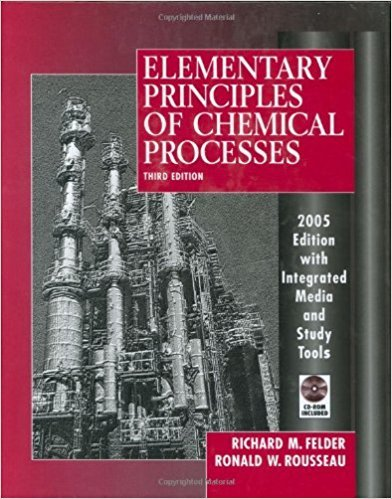×
Get Full Access to Elementary Principles Of Chemical Processes - 3 Edition - Chapter 8 - Problem 8.95
Get Full Access to Elementary Principles Of Chemical Processes - 3 Edition - Chapter 8 - Problem 8.95

×

# An aqueous solution containing 85.0 wt% H2S04 at 60FISBN: 9780471687573 143

## Solution for problem 8.95 Chapter 8

Elementary Principles of Chemical Processes | 3rd Edition

• Textbook Solutions
• 2901 Step-by-step solutions solved by professors and subject experts
• Get 24/7 help from StudySoup virtual teaching assistantsElementary Principles of Chemical Processes | 3rd Edition

4 5 1 261 Reviews
18
5
Problem 8.95

An aqueous solution containing 85.0 wt% H2S04 at 60F (specific gravity = 1.78) is diluted with pure liquid water at the same temperature. The feed solution volume is 350 mL. The mixing may be considered adiabatic, and the pressure is constant at 1 atm. (a) The product solution is to contain 30.0 wt% H2S04 . Calculate the volume (mL) of water needed for the dilution, ideally using a single-dimensional equation. (b) Use the enthalpy-concentration chart of Figure 8.5-1 to estimate the specific enthalpies (Btu/lbm) of the feed solution and the water. Then write an energy balance on this closed system constantpressure process and solve it for the specific enthalpy of the product solution. Finally, use Figure 8.5-1 to verify your calculated value ofIiproduct and to estimate the product solution temperature. (See Example 8.5-3.) (c) Use Figure 8.5-1 to estimate the maximum temperature that could be attained by mixing the feed solution with pure water and the concentration (wt% H2S04) of the product solution. (d) Good laboratory practice calls for adding acid to water when carrying out dilutions rather than vice versa. Use Figure 8.5-1 to justify this rule for the dilution of the feed solution in this problem

Step-by-Step Solution:
Step 1 of 3

Chapter 4  Stoichiometry The balanced equation for a reaction will tell you the relative numbers of the reactants and products, but in the lab we can't count out molecules to do a reaction, we can only weigh out the masses of the reactants and products. The following method can be used to calculate the amounts (in grams) of reactants needed and the amounts (in grams) of products that could be formed.  Calculating Quantities in a Reaction Equation- NH3 + O2 N2 + H2O Balanced Equation- 4 NH3 + 3 O2 2 N2 + 6 H2O The first step is to balance the equation. Put in the known quantity and indicate what you are trying to find. (grams, moles, or molar mass). 80grams to 4NH3 Calculate the molar mass of NH3. Molar mass of NH3

Step 2 of 3

Step 3 of 3

##### ISBN: 9780471687573

The full step-by-step solution to problem: 8.95 from chapter: 8 was answered by , our top Chemistry solution expert on 11/15/17, 02:42PM. Since the solution to 8.95 from 8 chapter was answered, more than 251 students have viewed the full step-by-step answer. The answer to “An aqueous solution containing 85.0 wt% H2S04 at 60F (specific gravity = 1.78) is diluted with pure liquid water at the same temperature. The feed solution volume is 350 mL. The mixing may be considered adiabatic, and the pressure is constant at 1 atm. (a) The product solution is to contain 30.0 wt% H2S04 . Calculate the volume (mL) of water needed for the dilution, ideally using a single-dimensional equation. (b) Use the enthalpy-concentration chart of Figure 8.5-1 to estimate the specific enthalpies (Btu/lbm) of the feed solution and the water. Then write an energy balance on this closed system constantpressure process and solve it for the specific enthalpy of the product solution. Finally, use Figure 8.5-1 to verify your calculated value ofIiproduct and to estimate the product solution temperature. (See Example 8.5-3.) (c) Use Figure 8.5-1 to estimate the maximum temperature that could be attained by mixing the feed solution with pure water and the concentration (wt% H2S04) of the product solution. (d) Good laboratory practice calls for adding acid to water when carrying out dilutions rather than vice versa. Use Figure 8.5-1 to justify this rule for the dilution of the feed solution in this problem” is broken down into a number of easy to follow steps, and 198 words. Elementary Principles of Chemical Processes was written by and is associated to the ISBN: 9780471687573. This full solution covers the following key subjects: solution, Water, feed, use, Product. This expansive textbook survival guide covers 13 chapters, and 710 solutions. This textbook survival guide was created for the textbook: Elementary Principles of Chemical Processes, edition: 3.

Unlock Textbook Solution